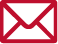HOME# Real-time optimum control design using Maple Non-Linear Model Predictive Control Design

### Do you have issues like these?I want to deal with a non-linear optimum control problem but am unsure of the analysis method to employ.The algorithms are difficult and it takes time to create programs.I want to perform real-time optimum control but the calculations involved are extensive and take a long time.

## Solution

### Overview

Non-linear model predictive control solves the problem of optimum control up to the finite-time future in each instant of time, and is a form of state feedback control that determines the control input. While deriving the optimal solution and performing feedback control in real-time is regarded as difficult to do realistically, using a high-speed solution known as the C/GMRES methodEwhich combines the continuous deformation method with the GMRES, real-time control has become possible. CYBERNET SYSTEMS has developed a non-linear model predictive control design tool using Maple, a software package supervised by Kyoto University professor Toshiyuki Ohtsuka.A simulation program is automatically generated in the C language based on state equations and evaluation functions given in Maple format. Then, the solution is processed at once from compilation to calculation results on the Maple worksheet.

## Advantages of the Solution

By using a non-linear model predictive control design worksheet through Maple, which utilizes the C/GMRES method, the following effects are expected.

• Non-linear optimum control problem analysis can be performed by simply setting the parameters depending on the problem
• Automation of cumbersome differential calculations and reduced labor for problem development
• Results of calculations can be displayed in a short time due to the use of high-speed algorithms

## Overview of Analysis Using the Solution

### Problem setting and parameter input

State equations, evaluation functions and parameters are set.### Maple-based automatic code generation

Maple formula manipulation is used to calculate the necessary functions and simplify the equations, and a C-language simulation program is automatically created.### C compiler execution and display of results

The generated C-language simulation problem is compiled and executed, and the calculation results are displayed. Either Microsoft Visual C++ compiler or GNU gcc compiler needs to be set as the C compiler.## Example: Hovercraft Position Control### Hovercraft materials (Ohtsuka Lab.)

http://www.symlab.sys.i.kyoto-u.ac.jp/~ohtsuka/research_j.htm
Videos of the hovercraft experiments are presented on this webpage.

## Required Products

• C compiler environment: Visual Studio C++ compiler or GNU gcc compiler
• OS environment: 64-bit Windows
• Maple version 17 or later recommended.

## InquiriesPlease direct any inquiries regarding Multi-domain Solutions to the following address.